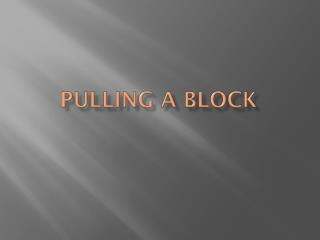DownloadDownload PresentationPulling a block

# Pulling a block

Download Presentation## Pulling a block

- - - - - - - - - - - - - - - - - - - - - - - - - - - E N D - - - - - - - - - - - - - - - - - - - - - - - - - - -
##### Presentation Transcript

1. Pulling a block

2. Pulling a Block

3. Pulling a block A 2.60 kg mass is being pulled by a force of 19.6 N at an angle of elevation of 35.0° as shown in the diagram below. The coefficient of friction between the floor and the block is 0.270. If the block starts from rest, what is its speed after being pulled with this force for 11.0 s? Hint: find the net acceleration first. (23 marks)

4. Pulling a Block • In order to solve the acceleration question, one must follow these steps: • Draw a free-body diagram. • Find the x- and y-components of the applied force vector. • Find the net force in the y-direction by adding the applied force in the y-direction to the weight if it’s being pushed and subtract the applied force in the y-direction if it’s being pulled. • Use the net force in the y-direction to calculate the force of friction. • Calculate the net force in the x-direction by subtracting the frictional force from the applied force in the x-direction. • Calculate the net acceleration by dividing the net force in the x-direction by the mass of the block. • Calculate final velocity using kinematics equations.

5. Ff Fapp Fappx Pulling a Block Fg To understand what is happening in this problem, one must first construct a free-body diagram to describe the forces involved. Fapp is the applied force acting on the mass. Fappx is the component of the applied force acting in the x-direction. Fappy Fappy is the component of the applied force acting in the y-direction. Fg is the force of gravity (weight) acting on the mass. Ff is the force of friction acting on the mass.

6. Fapp Pushing a Block In order to solve for the final speed of the block, we must first break down the applied force into its x- and y- components. Because this block is being pulled, the force acting in the y-direction is an upward force as seen here. If the block were being pushed, the force in the y-direction would be a downward force and it would add to the weight of the object being pushed. Fappy Fapp Fappy

7. Fapp Fappx Pulling a Block There is a component of the applied force that acts in the direction of motion, or the x-direction. We calculate this force by using the cos function. This component in the x-direction will be opposed by the force of friction. However, what must first be found is the net force acting in the y-direction.

8. Ff Fapp Pulling a Block Fg It is necessary for us to calculate the effective weight (net force acting in the y-direction) of the block, given the fact that it has its own weight MINUS the component of the pulling force that we have applied. Fappy The effective weight is increased when we are pushing because we are adding to the weight, but it is decreased when we are pulling the mass because we out y-component applies a lifting force to the mass. Fappx

9. Pulling a Block Fg It is necessary for us to calculate the effective weight (net force acting in the y-direction) of the block, given the fact that it has its own weight MINUS the component of the pulling force that we have applied. Fappy The effective weight is increased when we are pushing because we are adding to the weight, but it is decreased when we are pulling the mass because we out y-component applies a lifting force to the mass. Fnety

10. Ff Fapp Fappx Pulling a Block Once you have calculated the net force in the y-direction, you can then calculate the net force acting in the x-direction because the net force in the y-direction is needed to calculate the force of friction. Fappy One must finally calculate the net acceleration of the block using Newton’s 2nd Law.

11. Pulling a Block Once you have calculated the net force in the y-direction, you can then calculate the net force acting in the x-direction because the net force in the y-direction is needed to calculate the force of friction. One must finally calculate the net acceleration of the block using Newton’s 2nd Law. Fappx Fnetx Ff

12. Fapp Pulling a Block At this point the question merely becomes a kinematic question. We solve for the final velocity beginning with the definition of acceleration.